Ex 3.2

Chapter 3 Class 11 Trigonometric Functions
Serial order wise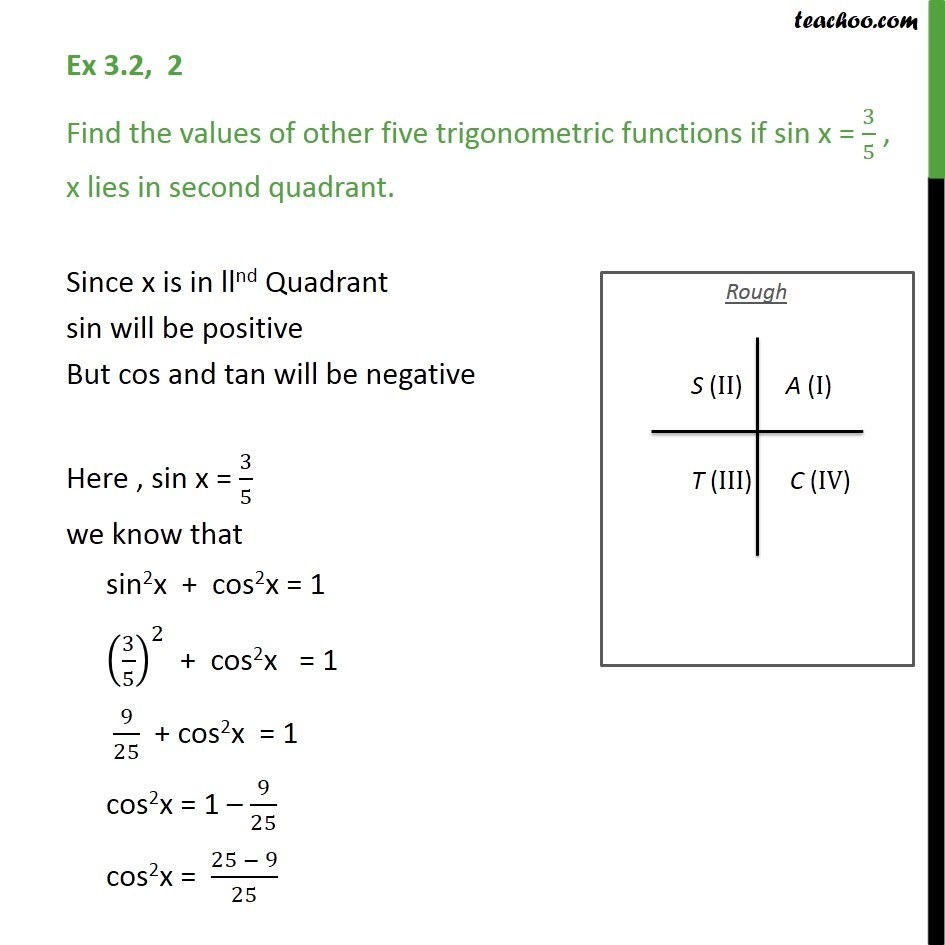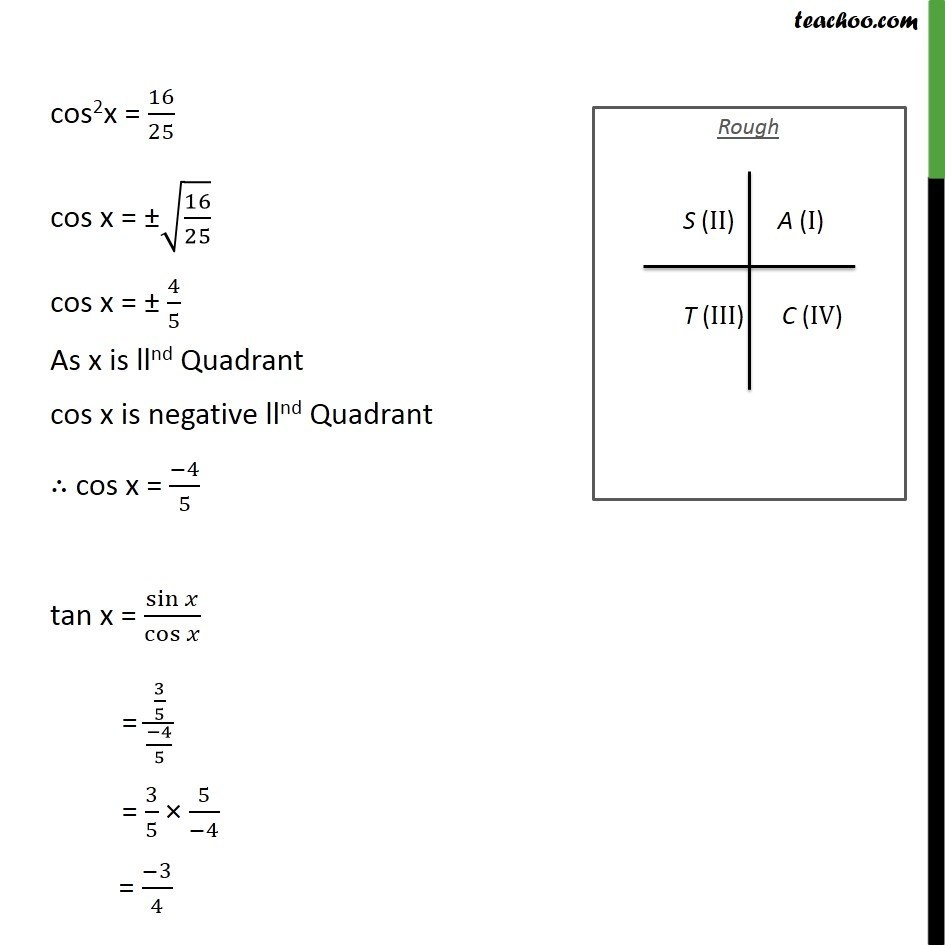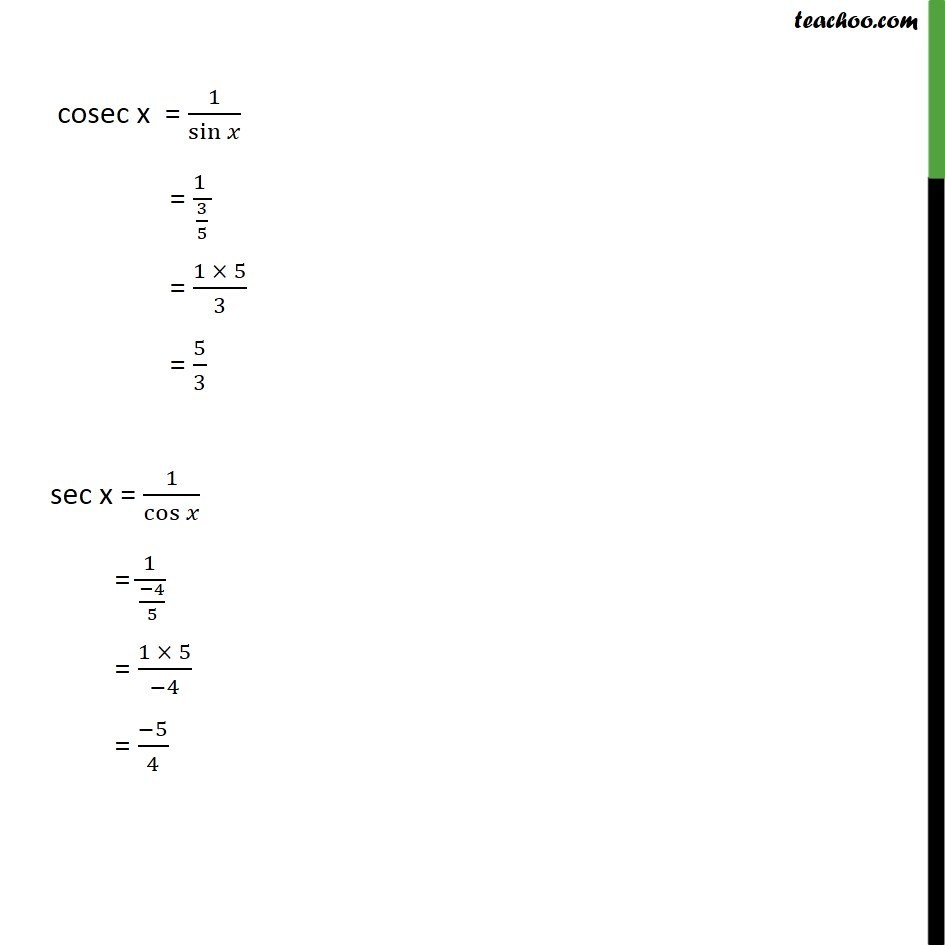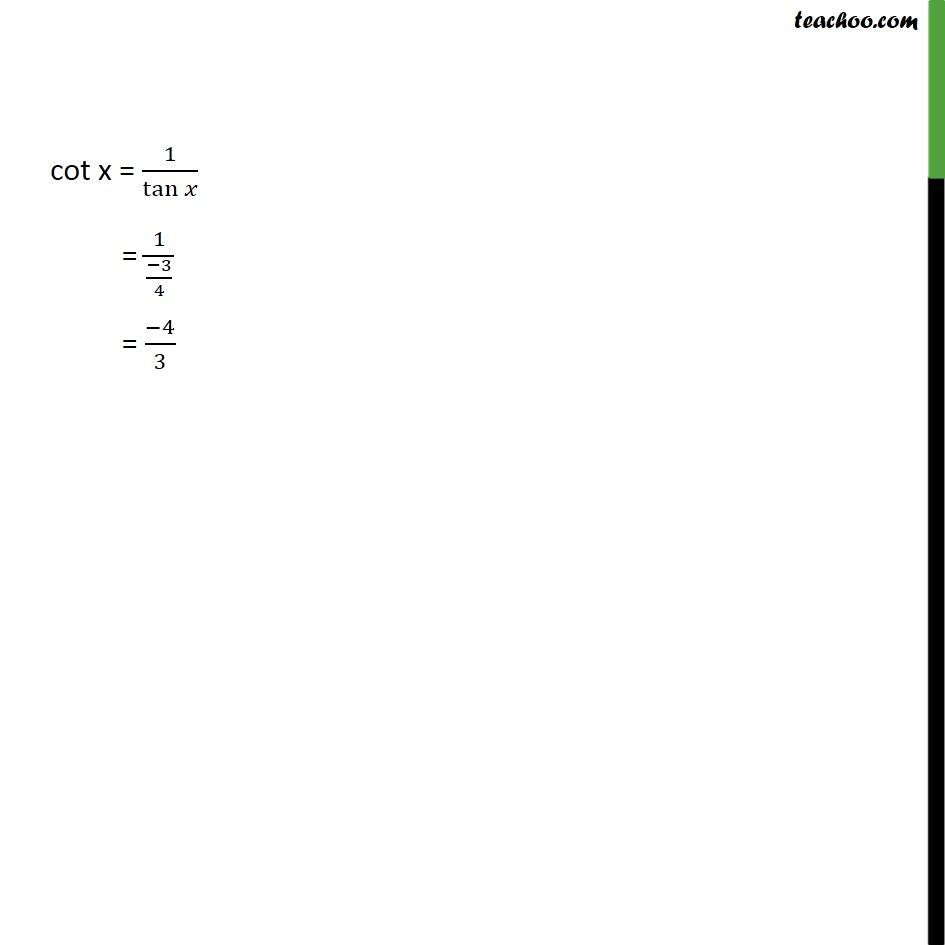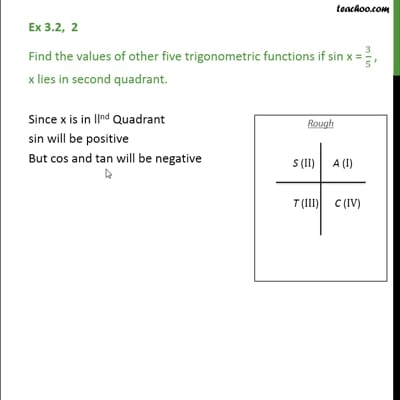This video is only available for Teachoo black users

Solve all your doubts with Teachoo Black (new monthly pack available now!)

### Transcript

Ex 3.2, 2 Find the values of other five trigonometric functions if sin x = 3/5 , x lies in second quadrant. Since x is in llnd Quadrant sin will be positive But cos and tan will be negative Here , sin x = 3/5 we know that sin2x + cos2x = 1 (3/5)^2 + cos2x = 1 9/25 + cos2x = 1 cos2x = 1 9/25 cos2x = (25 9)/25 cos2x = 16/25 cos x = (16/25) cos x = 4/5 As x is llnd Quadrant cos x is negative llnd Quadrant cos x = ( 4)/5 tan x = sin /cos = (3/5)/(( 4)/5) = 3/5 5/( 4) = ( 3)/4 cosec x = 1/sin = (1 )/(3/5) = (1 5)/3 = 5/3 sec x = 1/cos = (1 )/(( 4)/5) = (1 5)/( 4) = ( 5)/4 cot x = 1/tan = 1/(( 3)/4) = ( 4)/3